# ILP Part 37 — Send more money

This is the thirty seventh part of the ILP series. For your convenience you can find other parts in the table of contents in Part 1 – Boolean algebra

Today we are going to solve “Send more money” problem. We have the following addition: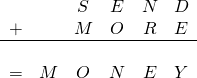Each letter represents exactly one digit in ten-based system. We need to find out numbers.
You can try to solve this on a piece of paper. The solution is;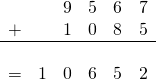We can solve this in ILP in at least two different ways. Let’s go.

# First solution

The most obvious way is to define digit variables, make sure, that they are different and that the first digit of a number is not zero: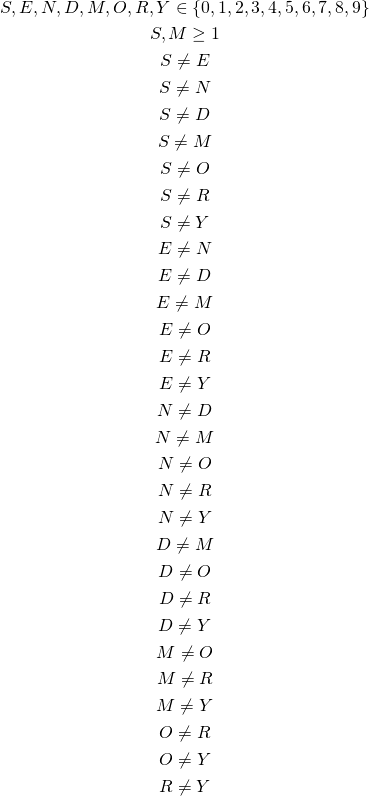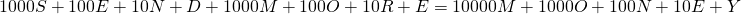This approach leads us right to the solution. Easy.

# Second solution

We can do this the long way by simulating long addition. We define variables in the same way as in previous solution, however, this time we add digits one by one. First, we add lowest digits: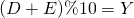We add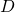and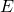, divide the result by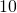and calculate the remainder. This remainder must be equal to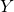.
Next, we add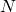and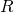. This time we need to include carry: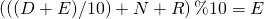We carry on with this method to calculate other digits: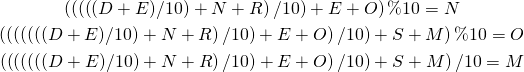This leads to the same solution, however, it is harder to read and a bit slower.

# Summary

As we can see, quite often there are multiple ways to represent calculations in ILP. Generally we should stick to more declarative approach and just define the problem as in first solution, however, sometimes we are able to rewrite imperative solution almost with no changes to linear problems. In next few parts we will see how to implement more imperative constructs: we will implement basic data structure, use it to simulate non-deterministic Turing machine, and finally solve even more sophisticated riddles.